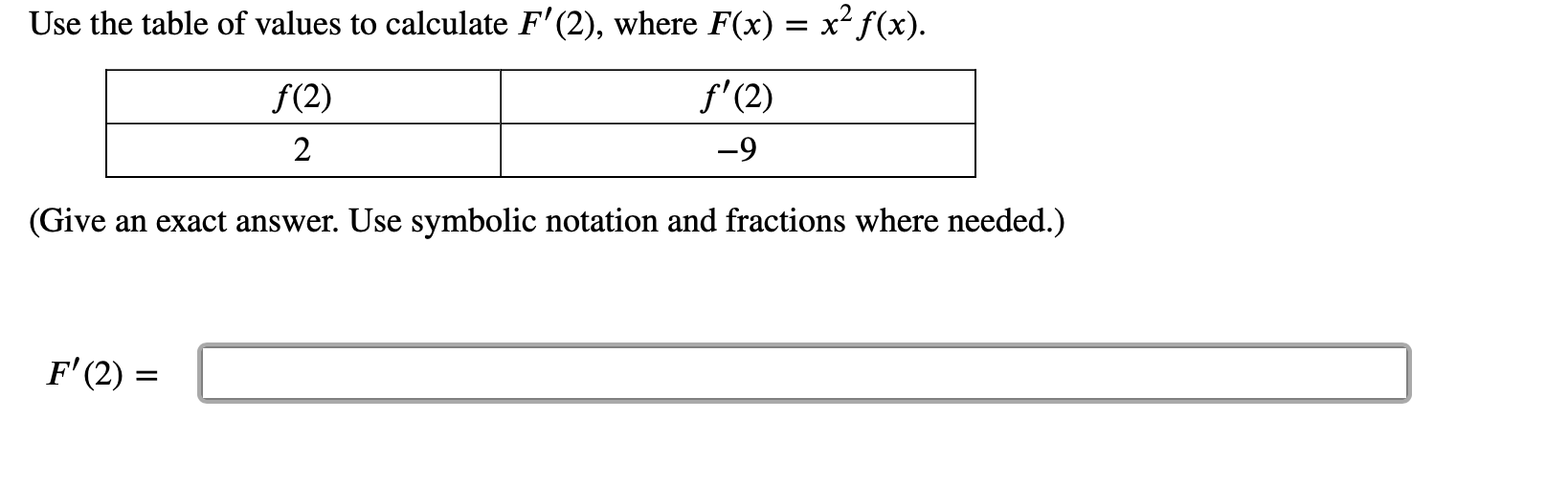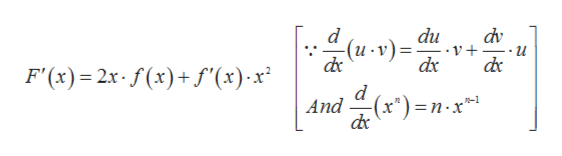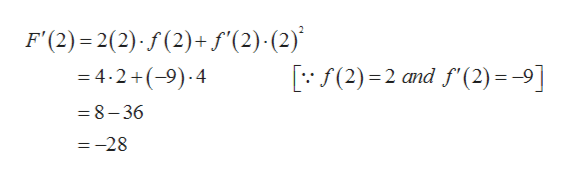# Use the table of values to calculate F'(2), where F(x) = x2f(x)f'(2)f(2)2-9(Give an exact answer. Use symbolic notation and fractions where needed.)F'(2)

Question
1 viewshelp_outlineImage TranscriptioncloseUse the table of values to calculate F'(2), where F(x) = x2f(x) f'(2) f(2) 2 -9 (Give an exact answer. Use symbolic notation and fractions where needed.) F'(2) fullscreen
check_circle

Step 1

Computing the derivative of F(x) by using product rule, we gethelp_outlineImage Transcriptionclosedu V+ dx d dv (u v)= F'(x) 2x f(x)f'(x)x d And (x") n x* dc R-1 fullscreen
Step 2

Now substituting x = 2 and ...help_outlineImage TranscriptioncloseF'(2) 2(2) (2)+ f'(2). (2) - 4.2+(-9) 4 (2) 2 nd f2) 9] = 8-36 =-28 fullscreen

### Want to see the full answer?

See Solution

#### Want to see this answer and more?

Solutions are written by subject experts who are available 24/7. Questions are typically answered within 1 hour.*

See Solution
*Response times may vary by subject and question.
Tagged in

### Calculus